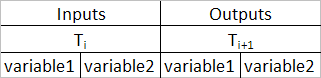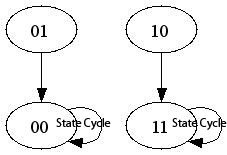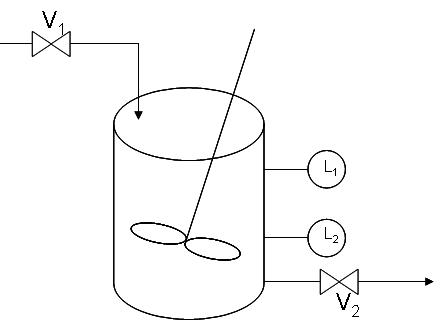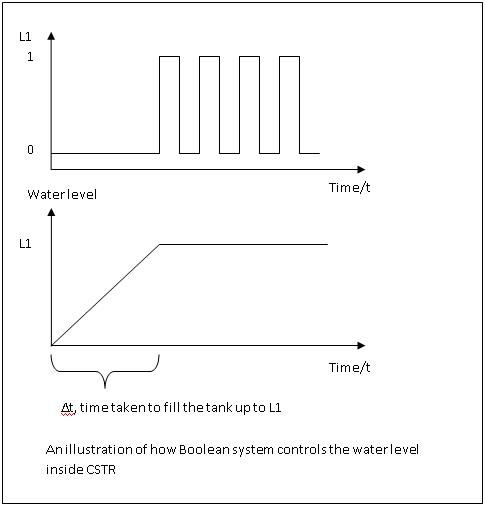# 5.1: Boolean Models - Truth Tables and State Transition Diagrams

•• Contributed by Peter Woolf et al.
• Assistant Professor (Chemical Engineering) at University of Michigan

Authors: Joseph Casler, Andry Haryanto, Seth Kahle and Weiyin Xu
Stewards: (September 12, 2007) Adhi Paisoseputra, Andrew Kim, Hillary Kast, Stephanie Cleto

## 1.1 Introduction to Boolean Models

A Boolean is a variable that can only attain two values: True or False. In most applications, it is convenient to represent a True by the number 1, and a False by the number 0. A Boolean model, or Boolean network, is a collection of Boolean variables that are related by logical switching rules, or Boolean functions, that follow an If-Then format. This type of Boolean model is known as an autonomous model and will be the primary type of model discussed in this article.

In chemical engineering, Boolean models can be used to model simple control systems. Boolean functions can be used to model switches on pumps and valves that react to readings from sensors that help keep that system operating smoothly and safely.

A simple application for level control of a CSTR is included in worked-out example 1. In this example, Boolean function is used to close the inlet stream and open the outlet stream when the level is higher than a specified point.

### 1.1.1 Boolean Functions

Boolean functions are logical operators that relate two or more Boolean variables within a system and return a true or false. A Boolean expression is a group of Boolean functions, which will be described individually below. When computing the value of a Boolean expression, Parentheses are used to indicate priority (working from inside out as in algebra). After that, LOGICAL INVERSION will always be first and LOGICAL EQUIVALENCE will be last, but the order of operation for the AND, OR, and EXCLUSIVE OR functions are specified with parenthesis.Descriptions and examples of these functions are given below. A quick reference of each of the functions can be found after the examples.

LOGICAL INVERSION

LOGICAL INVERSION is a function that returns the opposite value of a variable. The function is denoted as a prime on the variable (e.g. A' or B') For example, if we say that A is true (A=1), then the function A' will return a false (A'=0). Similarly, if we say that A is false (A=0) then the function A' will return true (A'=1).

Example:
A=1, B=A' then B=0

AND

The AND function relates two or more Boolean variables and returns a true if-and-only-if both variables are true. A dot is used to denote the AND function, or it is simply omitted. For example "A and B" can be written as "A•B" or as "AB." In this example, the AND function will only return a true if-and-only-if both Boolean variables A and B have a value of 1.

Examples:

 Variables Results A=1, B=1 AB = 1 A=1, B=0 AB = 0 A=1, B=1, C=1 ABC = 1 A=1, B=0, C=1 ABC = 0

OR

The OR function relates two or more Boolean variables and returns a true if any referenced variables are true. A plus is used to denote the OR function. For example "A or B" can be written as "A+B." In this example, the OR function will return true if either Boolean variable, A or B, has a value of 1.

Examples:

 Variables Results A=1, B=1 A+B = 1 A=1, B=0 A+B = 1 A=0, B=0 A+B = 0 A=0, B=0, C=1 A+B+C = 1 A=0, B=0, C=0 A+B+C = 0

EXCLUSIVE OR

The EXCLUSIVE OR function relates two or more Boolean variables and returns true only when one of the variables is true and all other variables are false. It returns false when more than one of the variables are true, or all the variables are false. A circumscribed plus is used to denote the EXCLUSIVE OR function. For example "A EXCLUSIVE OR B" can be written as "AB."

Examples:

 Variables Results A=1, B=1 AB = 0 A=1, B=0 AB = 1 A=0, B=1 AB = 1 A=0, B=0 AB = 0 A=0, B=0, C=0 ABC = 0 A=1, B=0, C=0 ABC = 1 A=1, B=0, C=1 ABC = 0 A=1, B=1, C=1 ABC = 0

LOGICAL EQUIVALENCE

The LOGICAL EQUIVALENCE function equates two Boolean variables or expressions. The LOGICAL EQUIVALENCE function, denoted as =, assigns a Boolean variable a true or false depending on the value of the variable or expression that it is being equated with. For example, A LOGICAL EQUIVALENCE B can be written as A = B. In this example, the value of A will be assigned the value of B.

### 1.1.2 Boolean Networks

As stated in the introduction, a Boolean network is a system of boolean equations. In chemical engineering, Boolean networks are likely to be dependant on external inputs as a means of controlling a physical system. However, the following sections pertain mostly to synchronous autonomous systems. An autonomous system is one that is completely independent of external inputs. Every Boolean variable is dependent on the state of other Boolean variables in the system and no variable is controlled by an external input. A synchronous system is one that logical switching (the changing of Boolean variables) occurs simultaneously for all variables based on the values prior to the incidence of change.

Here is an example of an autonomous boolean network:

 Boolean Functions A = B+C' B = AC C = A'

## 1.2 Truth Tables

A truth table is a tabulation of all the possible states of a Boolean Model at different time frames. A simple truth table shows the potential initial states at time, Ti, and the corresponding subsequent states at time Ti+1, of a Boolean network. Truth tables can provide one with a clearer picture of how the rules apply and how they affect each situation. Hence, they help to ensure that each output only has one control statement so that the Boolean rules do not conflict with each other.

### 1.2.1 Constructing Truth Tables

1. Draw up a table with the appropriate number of columns for each variable; one for each input and output.
2. The left side of the column should contain all possible permutations of the input variables at time Ti. One method to accomplish this might be to list all possible combinations in ascending binary order.
3. The right side of the column should contain the corresponding outcome of the output variables at the subsequent time Ti+1. A generic example of this with 2 variables can be seen below:A quick way to check that you have all of the possible permutations is that there should be 2x possible permutations for X input variables.

### 1.2.2 Example of a Truth Table

The sample system we will be using is based on hydrogen fuel cell technology. The equation for the operation of hydrogen fuel cells is

$\ce{H2 + O2 -> H2O.}$

One aspect of Proton Exchange Membrane (PEM) fuel cells (a type of fuel cell) is that the performance of the fuel cell is highly dependent on the relative humidity of the system (if humidity rises too high, the fuel cell will flood and H2 and O2 will be unable to reach the cell. If humidity falls too low, the fuel cell will dry up and the performance will drop.) The task is to create a Boolean model for this simplified water management system.The system produces steam within the system, and there is a vent to release steam if the system becomes too saturated. In our system, we will assume that the inputs are stoichimetric and react completely. Also we will assume that pressure buildup from steam is negligible compared to the change in relative humidity. The only variable in question is the %relative humidity in the system.

• note: this is not how water management actually works in a fuel cell system, but it is a simple example.

A will represent the moisture controller response (0 indicates relative humidity or %RH < 80%, 1 indicates %RH >80%)
B will represent the valve status (0 is closed, 1 is open)

The corresponding Boolean functions for this model are given below (normally you would have to design these yourself to meet the criteria you desire):

A = A
B = A

For this example with 2 input variables, there are 22 = 4 possible permutations and 22 = 4 rows. The resultant permutations for the outputs are: For A where Y=1, the number of 0s and 1s are 2(Y-1)=2(1-1)=1. For B where Y=2, the number of 0s and 1s are 2(Y-1)=2(2-1)=2.

The resultant truth table is below:## 1.3 State Transition Diagrams

A state transition diagram is a graphical way of viewing truth tables. This is accomplished by looking at each individual initial state and its resultant state. The transition from one state to another is represented by an arrow. Then they are pieced together like a jigsaw puzzle until they fit in place. When one state leads to itself it simply points to itself. The following example is based on the truth table in the previous section.In this example, there are two state cycles. A state cycle is a combination of states around which the system continually enters and reenters. For a finite number of states, there will always exist at least one state cycle. A state cycle is also a pathway or a flowchart that shows the "decision making process" of a Boolean network. This feature is a direct result from two attributes of Boolean networks:
1. Finite number of states
2. Deterministic (there is a certain set of rules that determines the next state that will be entered)

In the example presented in the previous section, there were two state cycles. One advantage of state cycles is it easily allows you to see where your model will end up cycling and if there are any states that are not accounted for properly by your model. In the previous diagram, if the moisture controller indicated the humidity was below the set value, it would close the valve or hold the valve closed. If the moisture controller indicated that the humidity was above the set value, it would either open the valve or hold it open.

Consider this alternate system.In this example, the state cycle says that if the meter says that the humidity is below the set point it would cycle the vent valve open and closed. This would hurt the system and is not a desired outcome of the model.

For safety and functionality issues, a process control engineer would want to consider all possiblities in the design of any Boolean network modeling a real system.

## 1.4 Limitations of Boolean Networks

### 1.4.1 Advantages of Boolean Networks

• Unlike ordinary differential equations and most other models, Boolean networks do not require an input of parameters.
• Boolean models are quick and easy to compute using computers.
• Boolean networks can be used to model a wide variety of activities and events.
• Boolean networks can be used to approximate ordinary differential equations when there are an infinite number of states.

### 1.4.2 Disadvantages of Boolean Networks

• Boolean networks are restrained to computing very simple math. They cannot be used for calculus and to calculate large quantities.
• Boolean models have relatively low resolution compared to other models.
• It is very time consuming and complicated to build Boolean networks by hand.

## 1.5 Examples

### 1.5.1 Worked out Example 1

A hypothetical CSTR needs to have its liquid level maintained below a safety mark by means of a sensor, L1, on the corresponding mark and a control valve placed on the inlet and outlet streams – V1 and V2 respectively. A typical application of the afore-mentioned system could involve heterogeneously catalyzed liquid reaction(s) with liquid product(s).Solution to Example 1

Conventions

Water level sensor

 L1 0 1 water level desirable too high

Valve

 V 0 1 position closed open

Initial State

Assume that the CSTR is empty and being filled up. CSTR, being empty, sets the value of L1 to zero. Filling up the CSTR could be done by opening valve 1 - V1 assuming a value of one - and closing valve 2 - V2 assuming a value of zero.

In coordinate form, the initial state is as such: (L1, V1, V2) = (0, 1, 0)

Problem Interpretation

Let h be the water level and WL1 be the safety mark defined in the CSTR. The system could assume one of the following states at any one time:

1) h < WL1 : desirable water level

Maximizing production of the chemical prompts the system to remain in its current state - that is, its initial state. (L1, V1, V2)final = (0, 1, 0) final state

2) h > WL1 : water level too high

Prevention of flooding requires that the tank be emptied. As such, valve 1 (V1) should be closed to stop the input while valve 2 (V2) should be open to empty the extra water above the safety water mark. (L1, V1, V2)' = (1, 1, 0) trigger to valve (L1, V1, V2)final = (1, 0, 1) final state

State CyclePhysical Significance## 1.6 Quick Reference